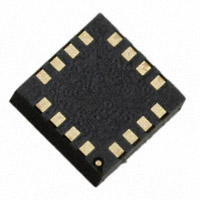< id="6e2kg">< id="6e2kg">< id="6e2kg">< id="6e2kg">< id="6e2kg">< id="6e2kg">< id="6e2kg">< id="6e2kg">< id="6e2kg">< id="6e2kg">< id="6e2kg">< id="6e2kg">< id="6e2kg">< id="6e2kg">< id="6e2kg">< id="6e2kg">< id="6e2kg">< id="6e2kg">< id="6e2kg">< id="6e2kg">< id="6e2kg">< id="6e2kg">< id="6e2kg">< id="6e2kg">< id="6e2kg">< id="6e2kg">< id="6e2kg">< id="6e2kg">< id="6e2kg">< id="6e2kg">< id="6e2kg">< id="6e2kg">< id="6e2kg">< id="6e2kg">< id="6e2kg">< id="6e2kg">< id="6e2kg">< id="6e2kg">< id="6e2kg">< id="6e2kg">< id="6e2kg">< id="6e2kg">< id="6e2kg">< id="6e2kg">< id="6e2kg">< id="6e2kg">< id="6e2kg">< id="6e2kg">< id="6e2kg">< id="6e2kg"> < id="6e2kg">< id="6e2kg">< id="6e2kg">< id="6e2kg">< id="6e2kg">< id="6e2kg">< id="6e2kg">< id="6e2kg">< id="6e2kg">< id="6e2kg">< id="6e2kg">< id="6e2kg">< id="6e2kg">< id="6e2kg">< id="6e2kg">< id="6e2kg">< id="6e2kg">< id="6e2kg">< id="6e2kg">< id="6e2kg">< id="6e2kg">< id="6e2kg">< id="6e2kg">< id="6e2kg">< id="6e2kg">< id="6e2kg">< id="6e2kg">< id="6e2kg">< id="6e2kg">< id="6e2kg">< id="6e2kg">< id="6e2kg">< id="6e2kg">< id="6e2kg">< id="6e2kg">< id="6e2kg">< id="6e2kg">< id="6e2kg">< id="6e2kg">< id="6e2kg">< id="6e2kg">< id="6e2kg">< id="6e2kg">< id="6e2kg">< id="6e2kg">< id="6e2kg">< id="6e2kg">< id="6e2kg">< id="6e2kg">< id="6e2kg"> < id="6e2kg">< id="6e2kg">< id="6e2kg">< id="6e2kg">< id="6e2kg">< id="6e2kg">< id="6e2kg">< id="6e2kg">< id="6e2kg">< id="6e2kg">< id="6e2kg">< id="6e2kg">< id="6e2kg">< id="6e2kg">< id="6e2kg">< id="6e2kg">< id="6e2kg">< id="6e2kg">< id="6e2kg">< id="6e2kg">< id="6e2kg">< id="6e2kg">< id="6e2kg">< id="6e2kg">< id="6e2kg">< id="6e2kg">< id="6e2kg">< id="6e2kg">< id="6e2kg">< id="6e2kg">< id="6e2kg">< id="6e2kg">< id="6e2kg">< id="6e2kg">< id="6e2kg">< id="6e2kg">< id="6e2kg">< id="6e2kg">< id="6e2kg">< id="6e2kg">< id="6e2kg">< id="6e2kg">< id="6e2kg">< id="6e2kg">< id="6e2kg">< id="6e2kg">< id="6e2kg">< id="6e2kg">< id="6e2kg">< id="6e2kg">型号： HMC5883L-TR 厂商： Honeywell Microelectronics & Precision Sensors 标准：分类： 传感器，变送器 ， 磁性传感器 - 霍尔效应，数字开关，线性，罗盘 (IC) 描述： IC compass 3 axis i2c 16lcc smd PDF： 预览 报错 收藏 赞

Datasheet下载地址

 本地下载 >> 第三方平台下载 >>

HMC5883L-TR的详细信息

Datasheets： 4,000 Sensors, Transducers Magnetic Sensors - Hall Effect, Digital Switch, Linear, Compass (ICs) HMC Tape & Reel (TR) ±8G Compass - Three Axis 2.16 V ~ 3.6 V 100μA - I2C Compass - Three Axis -30°C ~ 85°C 16-LCC 16-LCC HMC5883L 342-1082-2HMC5883LTR
HMC5883L
Errata Sheet
During testing of the new HMC5883L parts, the following issues were identified:
1. The current consumption in all modes is 27 μA higher than specified in the HMC5883L datasheet.
2. In the event the ADC reading overflows or underflows for the given channel, or if there is a math
overflow during the measurement, the data register will contain the value 0 or half the expected value.
To ensure this condition is avoided, use a gain register setting that allows for sufficient dynamic field
Please note that these discrepancies are found in the parts you are receiving and will be corrected in the units
scheduled for Q1 2011.
FIND OUT MORE
For
more
information
on
Honeywell’s
Magnetic
Sensors
visit
us
online
at
technical support email us at magnetic-support.ssec@honeywell.com.
The application circuits herein constitute typical usage and interface of Honeywell product. Honeywell does not warranty or assume
liability of customer-designed circuits derived from this description or depiction.
Honeywell reserves the right to make changes to improve reliability, function or design. Honeywell does not assume any liability arising
out of the application or use of any product or circuit described herein; neither does it convey any license under its patent rights nor the
rights of others.
Honeywell
12001 Highway 55
Plymouth, MN 55441
Tel: 763-954-2474, 800-323-8295
www.honeywell.com/magneticsensors
July 2010
?2010 Honeywell International Inc.